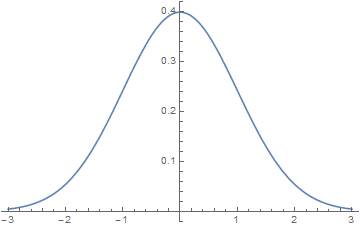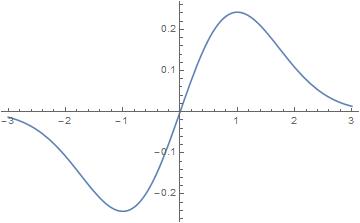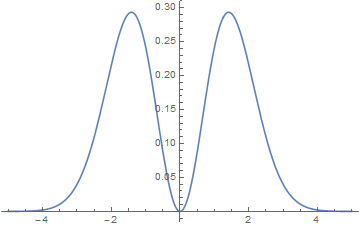20
Sep 16

## The pearls of AP Statistics 29

### Normal distributions: sometimes it is useful to breast the current

The usual way of defining normal variables is to introduce the whole family of normal distributions and then to say that the standard normal is a special member of this family. Here I show that, for didactic purposes, it is better to do the opposite.

### Standard normal distribution

The standard normal distribution$z$ is defined by its probability density$p(x)=\frac{1}{\sqrt{2\pi}}\exp(-\frac{x^2}{2}).$

Usually students don't remember this equation, and they don't need to. The point is to emphasize that this is a specific density, not a generic "bell shape".Figure 1. Standard normal density

From the plot of the density (Figure 1) they can guess that the mean of this variable is zero.Figure 2. Plot of xp(x)

Alternatively, they can look at the definition of the mean of a continuous random variable$Ez=\int_\infty^\infty xp(x)dx$. Here the function$f(x)=xp(x)$ has the shape given in Figure 2, where the positive area to the right of the origin exactly cancels out with the negative area to the left of the origin. Since an integral means the area under the function curve, it follows that

(1)$Ez=0.$

To find variance, we use the shortcut:$Var(z)=Ez^2-(Ez)^2=Ez^2=\int_{-\infty}^\infty x^2p(x)dx=2\int_0^\infty x^2p(x)dx=1.$Figure 3. Plot of x^2p(x)

The total area under the curve is twice the area to the right of the origin, see Figure 3. Here the last integral has been found using Mathematica. It follows that

(2)$\sigma(z)=\sqrt{Var(z)}=1.$

### General normal distribution

Fix some positive$\sigma$ and real$\mu$. A (general) normal variable$\mu$ is defined as a linear transformation of$z$:

(3)$X=\sigma z+\mu.$

Changing$\mu$ moves the density plot to the left (if$\mu$ is negative) and to the right (if$\mu$ is positive). Changing$\sigma$ makes the density peaked or flat. See video. Enjoy the Mathematica file.

Properties follow like from the horn of plenty:

A) Using (1) and (3) we easily find the mean of$X$:$EX=\sigma Ez+\mu=\mu.$

B) From (2) and (3) we have$Var(X)=Var(\sigma z)=\sigma^2Var(z)=\sigma^2$

(the constant$\mu$ does not affect variance and variance is homogeneous of degree 2).

C) Solving (3) for$z$ gives us the z-score:$z=\frac{X-\mu}{\sigma}.$

D) Moreover, we can prove that a linear transformation of a normal variable is normal. Indeed, let$X$ be defined by (3) and let$Y$ be its linear transformation:$Y=\delta X+\nu.$ Then$Y=\delta (\sigma z+\mu)+\nu=\delta\sigma z+(\delta\mu+\nu)$

is a linear transformation of the standard normal and is therefore normal.

Remarks. 1) In all of the above, no derivation is longer than one line. 2) Reliance on geometry improves understanding. 3) Only basic properties of means and variances are used. 4) With the traditional way of defining the normal distribution using the equation$p(x)=\frac{1}{\sqrt{2\pi\sigma^2}}\exp(-\frac{(x-\mu)^2}{2\sigma^2})$

there are two problems. Nobody understands this formula and it is difficult to extract properties of the normal variable from it.

Compare the above exposition with that of Agresti and Franklin: a) The normal distribution is symmetric, bell-shaped, and characterized by its mean μ and standard deviation σ (p.277) and b) The Standard Normal Distribution has Mean = 0 and Standard Deviation = 1 (p.285). It is the same old routine: remember this, remember that.